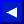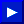Integer constants are whole numbers (numbers without decimal points). Integer constants must be between -32768 and +32767. Integer constants do not have decimal points or commas between digits. If the plus (+) sign is left out, the constant is assumed to be a positive number. Zeros coming before a constant are ignored and shouldn't be used since they waste memory and slow down your program. However, they won't cause an error. Integers are stored in memory as two-byte binary numbers. Some examples of integer constants are:

-12
8765
-32768
+44
0
-32767

 NOTE: Do NOT put commas inside any number. For example, always type 32,000 as 32000. If you put a comma in the middle of a number you will get the BASIC error message ?SYNTAX ERROR.

Floating-point constants are positive or negative numbers and can contain fractions. Fractional parts of a number may be shown using a decimal point. Once again remember that commas are NOT used between numbers. If the plus sign (+) is left off the front of a number, the Commodore 64 assumes that the number is positive. If you leave off the decimal point the computer will assume that it follows the last digit of the number. And as with integers, zeros that come before a constant are ignored. Floating-point constants can be used in two ways:

1. SIMPLE NUMBER
2. SCIENTIFIC NOTATION

Floating-point constants will show you up to nine digits on your screen. These digits can represent values between -999999999. and +999999999. If you enter more than nine digits the number will be rounded based on the tenth digit. if the tenth digit is greater than or equal to 5 the number will be rounded upward. Less than 5 the number be rounded downward. This could be important to the final totals of some numbers you may want to work with.

Floating-point numbers are stored (using five bytes of memory) and are manipulated in calculations with ten places of accuracy. However,This page has been created by Sami Rautiainen. Read the small print. Last updated November 13, 1998.